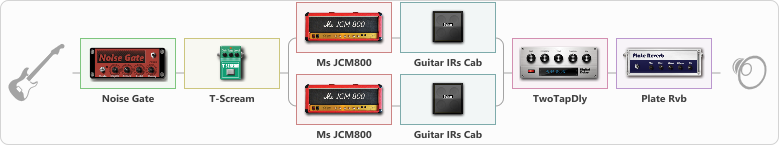# Riff variation

Discussion in 'ToneLib-GFX presets' started by tod, Feb 11, 2020.

1. Riff variation

Preset name: Riff variation

Cort KX500MS, bridge EMG humbacker

Effects chain:Effect: "Noise Gate" (Dynamics / Filter), active - "yes"
{
"Mode" = Manual
"Depth" = 46
"Threshold" = 50
"Attack" = 0
"Hold" = 5
"Decay" = 250
}

Effect: "T-Scream" (Overdrive / Distortion), active - "yes"
{
"Drive" = 23
"Tone" = 63
"Level" = 44
}

Effect: "Splitter" (Dynamics / Filter), active - "yes"
{
"A-Bypass" = Off
"A-Pan" = -35
"A-Level" = 55
"B-Bypass" = Off
"B-Pan" = 35
"B-Level" = 55

Left branch:
{

Effect: "Ms JCM800" (Amp simulators), active - "yes"
{
"Gain" = 85
"Bass" = 77
"Middle" = 0
"Treble" = 82
"Presence" = 41
"Master" = 77
"Level (dB)" = 0
}

Effect: "Guitar IRs Cab" (Cabinets), active - "yes"
{
"Model" = Marshall JCM2000 (4x12")
"Mic Position" = Middle
"Mic Distance" = Near
"Low Cut (Hz)" = 60
"Hi Cut (kHz)" = 20.0
"Mix" = 100
"Level (dB)" = 0
}
}
Right branch:
{

Effect: "Ms JCM800" (Amp simulators), active - "yes"
{
"Gain" = 85
"Bass" = 51
"Middle" = 100
"Treble" = 53
"Presence" = 41
"Master" = 77
"Level (dB)" = 0
}

Effect: "Guitar IRs Cab" (Cabinets), active - "yes"
{
"Model" = Marshall 1960A (4x12")
"Mic Position" = Middle
"Mic Distance" = Near
"Low Cut (Hz)" = 60
"Hi Cut (kHz)" = 20.0
"Mix" = 100
"Level (dB)" = 0
}
}
}

Effect: "TwoTapDly" (Delay), active - "yes"
{
"Time" = 42
"Feedback" = 0
"Tone" = 11
"Sens" = 0
"TapTime" = 8.9
"Mix" = 59
}

Effect: "Plate Rvb" (Reverb), active - "yes"
{
"Time" = 8.4
"PreDelay" = 41
"LoDamp" = 32
"HiDamp" = 17
"Mix" = 37
}

Note: You will need to download and install the ToneLib-GFX software to use the preset.

File size:
966 bytes
Views:
3,284